International
Tables for
Crystallography
Volume B
Reciprocal space
Edited by U. Shmueli

International Tables for Crystallography (2010). Vol. B, ch. 1.3, pp. 58-59   | 1 | 2 |

## Section 1.3.3.3.2.1. Multidimensional Cooley–Tukey factorization

G. Bricognea

aGlobal Phasing Ltd, Sheraton House, Suites 14–16, Castle Park, Cambridge CB3 0AX, England, and LURE, Bâtiment 209D, Université Paris-Sud, 91405 Orsay, France

#### 1.3.3.3.2.1. Multidimensional Cooley–Tukey factorization

| top | pdf |

Let us now assume that this decimation can be factored into d successive decimations, i.e. thatand henceThen the coset decomposition formulae corresponding to these successive decimations (Section 1.3.2.7.1) can be combined as follows: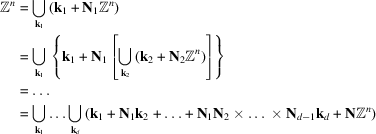with. Therefore, anymay be written uniquely asSimilarly: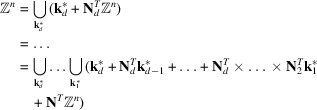so that anymay be written uniquely aswith. These decompositions are the vector analogues of the multi-radix number representation systems used in the Cooley–Tukey factorization.

We may then write the definition ofwithfactors as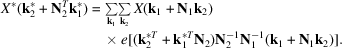The argument of e(–) may be expanded asThe first summand may be recognized as a twiddle factor, the second and third as the kernels ofand, respectively, while the fourth is an integer which may be dropped. We are thus led to a vector-radix' version of the Cooley–Tukey algorithm, in which the successive decimations may be introduced in all n dimensions simultaneously by general integer matrices. The computation may be decomposed into five stages analogous to those of the one-dimensional algorithm of Section 1.3.3.2.1:

 (i) form thevectorsof shapeby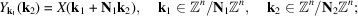(ii) calculate thetransformsonpoints: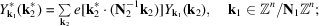(iii) form thevectorsof shapeby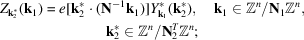(iv) calculate thetransformsonpoints: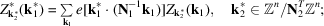(v) collectas.

The initial-point transformcan thus be performed astransformsonpoints, followed bytransformsonpoints. This process can be applied successively to all d factors. The same decomposition applies to, up to the complex conjugation of twiddle factors, the normalization factorbeing obtained as the product of the factorsin the successive partial transforms.

The geometric interpretation of this factorization in terms of partial transforms on translates of sublattices applies in full to this n-dimensional setting; in particular, the twiddle factors are seen to be related to the residual translations which place the sublattices in register within the big lattice. If the intermediate transforms are performed in place, then the quantitywill eventually be found at locationso that the final results will have to be unscrambled by a process which may be called coset reversal', the vector equivalent of digit reversal.

Factoring by 2 in all n dimensions simultaneously, i.e. taking, leads to n-dimensional butterflies'. Decimation in time corresponds to the choice, so thatis an n-dimensional parity class; the calculation then proceeds by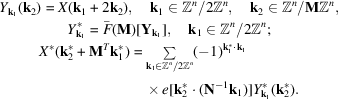Decimation in frequency corresponds to the choice,, so thatlabels octant' blocks of shape M; the calculation then proceeds through the following steps:i.e. theparity classes of results, corresponding to the different, are obtained separately. When the dimension n is 2 and the decimating matrix is diagonal, this analysis reduces to the `vector radix FFT' algorithms proposed by Rivard (1977)and Harris et al. (1977). These lead to substantial reductions in the number M of multiplications compared to the row–column method: M is reduced toby simultaneousfactoring, and toby simultaneousfactoring.

The use of a nondiagonal decimating matrix may bring savings in computing time if the spectrum of the band-limited function under study is of such a shape as to pack more compactly in a nonrectangular than in a rectangular lattice (Mersereau, 1979). If, for instance, the support K of the spectrum Φ is contained in a sphere, then a decimation matrix producing a close packing of these spheres will yield an aliasing-free DFT algorithm with fewer sample points than the standard algorithm using a rectangular lattice.

### References

Harris, D. B., McClellan, J. H., Chan, D. S. K. & Schuessler, H. W. (1977). Vector radix fast Fourier transform. Rec. 1977 IEEE Internat. Conf. Acoust. Speech Signal Process. pp. 548–551.
Mersereau, R. M. (1979). The processing of hexagonally sampled two-dimensional signals. Proc. IEEE, 67, 930–949.
Rivard, G. E. (1977). Direct fast Fourier transform of bivariate functions. IEEE Trans. Acoust. Speech Signal Process. 25, 250–252.